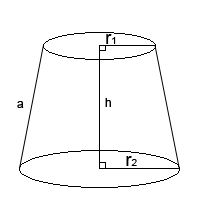## How to Calculate and Solve for the Total Surface Area of a Conical Frustum | The Calculator EncyclopediaThe image above is a conical frustum.

To compute the total surface area of a conical frustum requires three essential parameters which are the radius of the lower base (R), radius of the upper base (r) and the height (h).

The formula for calculating the total surface area of a conical frustum;

A = π[R² + r² + (R + r)√((R – r)² + h²)]

Where;
A = Total surface area of the conical frustum
R = Radius of the lower base
r = Radius of the upper base
h = Height of the conical frustum

Let’s solve an example;
Find the total surface area of the conical frustum when the radius of the upper base is 11 cm, radius of the lower base is 17 cm and the height is 30 cm.

This implies that;
r = Radius of the upper base = 11 cm
R = Radius of the lower base = 17 cm
h = Height of the conical frustum = 30 cm

A = π[R² + r² + (R + r)√((R – r)² + h²)]
A = π[17² + 11² + (17 + 11)√((17 – 11)² + 30²)]
A = π[289 + 121 + (28)√((6)² + 900)]
A = π[289 + 121 + (28)√(36+ 900)]
A = π[289 + 121 + (28)√(936)]
A = π[289 + 121 + (28)(30.59)]
A = π[289 + 121 + 856.63]
A = π[1266.63]
A = 3979.25

Therefore, the total surface area of the conical frustum is 3979.25 cm².